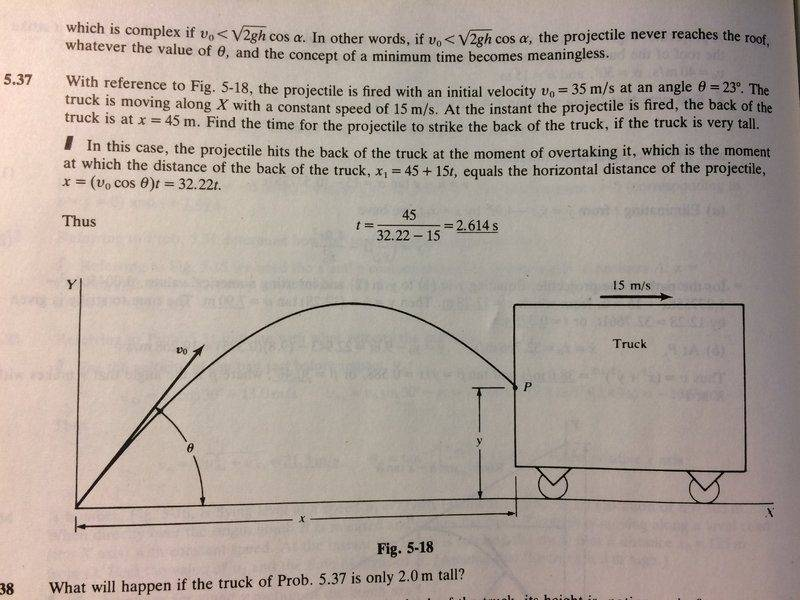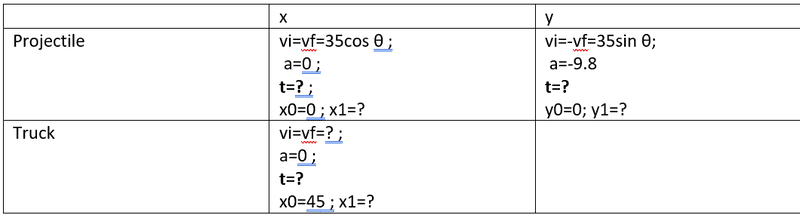# Projectile Motion: time it takes for projectile to hit truck

• Beth N

## Homework Statement

Problem: 5. 37 [/B]
With reference to the figure below, the projectile is fired with an initial velocity ##v_0=35 m/s ## at θ=23°.
Truck is moving along X with constant speed of 15m/s
At instant projectile is fired, back of truck is at x=45m
Find time for the projectile to strike back of the truck if the truck is very tall.## Homework Equations

##x=x_0+v_0t+(\frac 1 2)at^2##
##v_{ave}=Δx/Δt ##
##v_x=vcosθ ## ; ##v_y=vsinθ ##

## The Attempt at a Solution

At first I thought that I can assume that the project tile hits the truck right at the position where y=0. My equation was:

##y-y_0=v_{0y}t+(\frac 1 2)at^2=0 ##
##t= \frac {-v_{0y} *2} {a} ##
##t= \frac {-35sin(23)*2} {-9.8} ##
##t=2.79 ##

The key is 2.614, which is less than 2.79, which means the projectile strikes the truck before it reaches the ground. The time it takes for the projectile to land the ground (and complete the curve) is greater than the time it takes to hit the truck, as the diagram indicate.
My 1st question: How can I be sure that it is so? What does the key mean by saying "at the moment of overtaking it"? I was stuck on the way I formulate an equation for the time, which was this.
## t_{time taken to strike truck}=t_{time taken for Δy=0} - t_{?? not sure how to determine} ##

It seems like I have an ineffective way of thinking through this problem, and I'm curious on what are better ways.

Unfortunately, it took me a long time (20 minutes for this one) to brainstorm the solution even though on tests I'm supposed to solve them in 3-5 minutes. I understand the concept of projectile motion, but cannot apply to problems. How can I be faster? I tried several methods including re-drawing the diagrams and putting variables in a table like below.My 2nd question : how to improve in general problem-solving in physics, specifically problems about kinematics. Thank you!

#### Attachments

At first I thought that I can assume that the project tile hits the truck right at the position where y=0.
Why assume that?

My 1st question: How can I be sure that it is so? What does the key mean by saying "at the moment of overtaking it"?
What matters is that the projectile has to catch up with the truck. That means the projectile and the back of the truck must be at the same position along the x-axis. (Thus the horizontal motion is key!)

My 2nd question : how to improve in general problem-solving in physics, specifically problems about kinematics.
Not to sound silly, but the secret is: Practice! Get yourself a problem book and get busy. The more problems you solve, the better you'll get.

•Beth N
Ask yourself. Does it matter how high the projectile reaches? yes or no answer.

•Beth N
My 1st question: How can I be sure that it is so?
You can compute how long it takes for the projectile to hit the truck and compare it to the time it would take the projectile to land. To assume delta y = 0 is not motivated. Anybody who got their windshield destroyed due to stone chips flying off the road should be able to testify to that.

In fact, you should also be able to compute where it hits.

The key is 2.614, which is less than 2.79,
Please always use units for dimensionful numbers! Also, with the given data, answering with anything more than two significant digits is not well motivated.

•Beth N
Thank you all! It makes sense now.

Not to sound silly, but the secret is: Practice! Get yourself a problem book and get busy. The more problems you solve, the better you'll get.
Thank you. I do need practice. Do you have any recommendation for a problem book? I'm looking for a college algebra-based problem book. I'm using Schaum's "3000 solved problems in Physics" right now but would appreciate a second opinion!

I've found "THE PHYSICS PROBLEM SOLVER" to be quite useful. ISBN 0878915079.
Publisher is: Research and Education Association, 61 Ethel Road West, Piscataway, New Jersey 08854

At over 1000 pgs it has enough problems to keep you busy, and it also has the detailed solution and explanation for each.
I have the 1988 soft cover printing but it was originally published in 1978, and was appearently available in 1995 as a hard cover.

I don't know if it is still in print but a search (https://www.google.com/search?&q=isbn+0878915079) finds many sellers from $4 to$55 for used and new.

Cheers,
Tom

•Beth N
Hi what about approaching the solution graphically. The truck can be represented by a straight line and the projectile follows a curved one. A point of intersection would represent the time of impact

•Beth N
Hi what about approaching the solution graphically. The truck can be represented by a straight line and the projectile follows a curved one. A point of intersection would represent the time of impact
No. The horizontal motion is linear in both cases. You could do this but both curves would be straight lines (of different slope).

Hi but it looks like one of two curves is parabolic (concave down) and there would be an eventual point of intersection. I don’t see how both equations are linear because one the two equations has a degree greater than 1. If the lines were straight and at different slopes they may or may not ever intersect. I they do not intersect that implies the projectile will never hit the truck. If there is a solution graphically that will provide the time to impact, distance traveled depending on how the equations are setup for the respective solution

Hi but it looks like one of two curves is parabolic (concave down) and there would be an eventual point of intersection. I don’t see how both equations are linear because one the two equations has a degree greater than 1. If the lines were straight and at different slopes they may or may not ever intersect. I they do not intersect that implies the projectile will never hit the truck. If there is a solution graphically that will provide the time to impact, distance traveled depending on how the equations are setup for the respective solution
The vertical motion of the projectile is irrelevant. [Re-read the OP -- the truck is "very tall"]. The horizontal motion of the projectile plotted as horizontal distance versus time is a straight-line graph. The position of the back of the truck plotted as horizontal distance versus time is also a straight line graph.

•Beth N
Hi but it looks like one of two curves is parabolic (concave down) and there would be an eventual point of intersection.
Those are not curves of distance vs time, they are curves of height vs distance (in which the truck is just a horizontal line).

The only relevance of the vertical motion is to confirm that the projectile is above ground at the point it overtakes the truck. If you solve for the height of the projectile at that point and the value is negative, that means that the projectile hit the ground before it could overtake the truck.•Beth N
Thank you. I do need practice. Do you have any recommendation for a problem book? I'm looking for a college algebra-based problem book. I'm using Schaum's "3000 solved problems in Physics" right now but would appreciate a second opinion!
I think that one's pretty good. Another option is to look for review books for the AP (Advanced Placement) Physics 1 exam. Even though it's a high school course, I've found it largely covers the same material as a college-level algebra-based physics course. And the "review" portions might be helpful since they pull out the key points for you.

When you practice working problems, resist the temptation to look at the solutions too soon. You have to struggle a bit for it to sink in. (By looking at the solutions too soon you might fool yourself into thinking you've got it since lots of things make sense when you see someone else doing it.) Other tips: Revisit any problems that proved difficult. Solve them again the following week. (Without looking anything up!) Also, be sure to mix things up: When practicing problems in your current chapter, be sure to toss in a few from previous chapters.

What textbook are you using?

Ok back to the figure the y-axis is the height of the projectile and truck relative to ground respectively. The x-axis is the horizontal distance covered by the projectile and truck. I don’t see an indicator for tallness but the the point “p” is an arbitrary height as shown on the diagram. Since this point looks midway, the tallness of the truck would be 2p So the problem appears to really be when does the projectile reach the height “p” for a truck gaining horizontal distance from the projectile. So for question #1 the assumption is the projectile is gaining horizontal distance to the right faster than the truck is moving in the same direction, Using your answer graph the truck and projectile and see if projectile is at the same point in horizontal distance. That will help you test your answer. Assuming the projectile is moving faster than the truck the instance in time when the projectile is equal to the height p and the same horizontal distance as the truck will be the point the projectile will overtake the truck. I think you need to look at the truck as a constraint equation to solve the problem

So the problem appears to really be when does the projectile reach the height “p” for a truck gaining horizontal distance from the projectile.
Since "p" is unknown, that won't help solve the problem. The correct way to approach the problem, as discussed above, is to first concentrate on the horizontal motion. Only after you've solved for when the projectile overtakes the truck would you even worry about the projectile's height "p".

•Tom.G and Orodruin